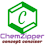Welcome to Chem Zipper.com......: BODY CENTERED CUBIC CELL (BCC):

## Thursday, April 25, 2019

### BODY CENTERED CUBIC CELL (BCC):

(1) A more efficiently packed cubic structure is the "body-centered cubic" (bcc).
(2) The first layer of a square array is expanded slightly in all directions. Then, the second layer is shifted so its spheres nestle in the spaces of the first layer.
(3) This repeating order of the layers is often symbolized as "ABA...".The considerable space shown between the spheres is misleading: spheres are closely packed in bcc solids and touch along the body diagonal.
(4) The packing efficiency of the bcc structure is about 68%. The coordination number for an atom in the bcc structure is eight.
(5) Both SCC and BCC are  not a closed packing in 3D.
(6) BCC crystal lattice is basically fusion of two simple unit cell in such that coner of one simple unit cell become body centred atom of another simple unit cell.
(7) Radius of atom and Packing efficiency in BBC unit cell:
Consider ‘r’ is  the radius of sphere and ‘a’ be the edge length of the cube and the sphere at the centre touches the sphere at the corner. Therefore body diagonal

Packing efficiency (PE): Percentage of space occupied by spheres

No. of spheres in bcc = 2
Volume of 2 spheres = 2´4/3pr3

Empty space (% Voids) = 100- 68= 32 %

(8)  Density of BCC unit cell:
(9) Coordination Numbers:
(1) The nearest neighbour distance is half of body diagonal (a) root 3/2 (along body diagonal) therefore coordination number for a given atom in BCC unit cell is 8.
(2) The next nearest neighbour are 6 at distance (a) Lattice parameter.
(3) 3rd neighbour (Next to Next nearest neighbour) are (12) at distance (a root 2) ( All corner along face diagonal in x,y and Z plane).

ILLUSTRATIVE EXAMPLE (1): An element has a body-centred cubic (bcc) structure with a cell edge of 288 pm. The density of the element is 7.2 g/cm3. How many atoms are present in 208 g of the element ?
SOLUTION:
ILLUSTRATIVE EXAMPLE (1): How many 'nearest' and 'next nearest' neighbours respectively does potassium have in b.c.c. lattice
SOLUTION: 8 and 6

1.2.3.4.